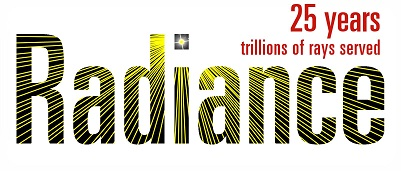# Cache point and ambient parameters

I just started using radiance and I think this is a very basic question.
I am exploring the relationship between -ad, -ab, -as, -aa, -ar and computational accuracy and computational load in order to find the appropriate parameter settings for lighting designers to simulate.
As a test model, we assume a simple building of 5m10m2.8m with only one window on the south side, and set the observation point at (2.5,5,0.7).
I set the above parameters appropriately, and tried to get a trend by looking at the number and location of sample points using lookamb.
At first, when I set ad = 2, ab = 1, which is a very small number of sample points, I assumed that the ray from the observation point would hit the surface of the room at 2^2 = 4 points, and that the information from 4 sample points would be output.
However, when ar=2, aa=0.5, the number of sample points was more than 4, about 8.
I assume this is due to the IC being done and the cache points being added.

In response to these, here are my questions.
[Q1]
What is the calculation algorithm for the ray being fired and the cache point being determined?
Here is a flowchart of what I am assuming. Is this correct? I’m sure it’s more complicated than that.

Q2.
When adding a new cache point by IC, how do you determine its position?

Q3]
What kind of algorithm is used for super sampling (-as)?
I imagine dividing the hemisphere that fires the ray into patches, and sending new rays between patches with large changes in brightness.

[Q4].
What is the u-vector output by lookamb?

The interreflection calculation is one of the most evolved parts of Radiance, and fairly complex as a result. The -ad and -as settings roughly control the initial samples for the first bounce, but the -ad setting is adjusted as needed to evenly divide a hemisphere.

[Q1]
What is the calculation algorithm for the ray being fired and the cache point being determined?
Here is a flowchart of what I am assuming. Is this correct? I’m sure it’s more complicated than that.

The first sample position (2.5, 5, 0.7) in your case causes the hemisphere of incident interreflections to be sampled, using at least 49 rays, which is the minimum if -aa > 0.
I did not see your flowchart. The code in rt/src/ambcomp.c is the ultimate reference.

[Q2]
When adding a new cache point by IC, how do you determine its position?

It is based on the accuracy estimate of nearby cached values, which depends on many things, including the Hessian calculation that determines indirect lighting gradients.

[Q3]
What kind of algorithm is used for super sampling (-as)?
I imagine dividing the hemisphere that fires the ray into patches, and sending new rays between patches with large changes in brightness.

Yes, it is something like that. Samples are added to hemisphere subdivisions based on stratified sampling and local variance estimates.

[Q4].
What is the u-vector output by lookamb?
This is a vector in the surface plane pointing in the direction of the maximum or minimum position gradient. (I don’t remember which.)

The genambpos.pl script in ray/src/util is a great way to visualize cache values, although it is not properly documented.

Best,
-Greg